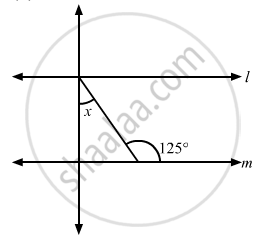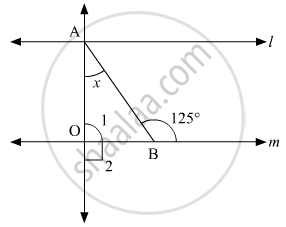Advertisement Remove all ads

# In the Given Figure, If Lines L and M Are Parallel, Then the Value of X is - Mathematics

MCQ

In the given figure, if lines l and m are parallel, then the value of x is#### Options

• 35°

• 55°

• 65°

• 75°

Advertisement Remove all ads

#### Solution

The given figure is as follows with : l || mAlso, ∠1 and ∠2 form a linear pair. Thus,

∠1 +∠2 = 180°

It is given that ∠2 = 90°, substituting this value , we get :

∠1 + 90° = 180°

∠1 = 180° - 190°

∠1 = 90°      (i)

In a triangle, we know that, the exterior angle is equal to the sum of the interior opposite angle.

In ΔAOB:

x + ∠1 = 125°

x = 125° - ∠1

From equation (i):

x = 125° - 90°

x = 35°

Is there an error in this question or solution?
Advertisement Remove all ads

#### APPEARS IN

RD Sharma Mathematics for Class 9
Chapter 10 Lines and Angles
Q 24 | Page 56
Advertisement Remove all ads

#### Video TutorialsVIEW ALL 

Advertisement Remove all ads
Share
Notifications

View all notifications

Forgot password?
Course